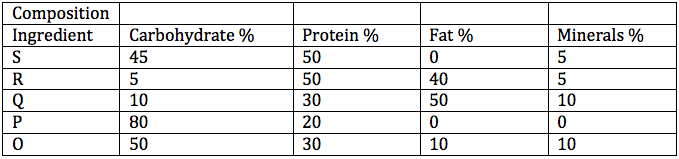# DI Practice Questions for CAT PDF

0
6810

Preparing for the Data Interpretation (DI) section of the CAT exam can be challenging, especially if you are new to this section. The best way to improve your DI skills is by practising with a variety of questions. To help you with your CAT exam preparation, we have compiled a set of DI practice questions for CAT with solutions in PDF format. In this article, we will provide an overview of the CAT DI section, the types of questions you can expect, and how these practice questions can help you prepare. These questions cover various topics and difficulty levels, giving you the opportunity to assess your proficiency and identify areas for improvement.

DI Practice Questions for CAT PDF:

Instructions:

Directions for the following four questions:
A health-drink company’s R&D department is trying to make various diet formulations, which can be used for certain specific purposes. It is considering a choice of 5 alternative ingredients (O, P, Q, R, and S), which can be used in different proportions in the formulations.

The table below gives the composition of these ingredients. The cost per unit of each of these ingredients is O: 150, P: 50, Q: 200, R: 500, S: 100.Question 1:

For a recuperating patient, the doctor recommended a diet containing 10% minerals and at least 30% protein. In how many different ways can we prepare this diet by mixing at least two ingredients?

a) One
b) Two
c) Three
d) Four
e) None

Question 2:

Which among the following is the formulation having the lowest cost per unit for a diet having 10% fat and at least 30% protein? The diet has to be formed by mixing two ingredients.

a) P and Q
b) P and S
c) P and R
d) Q and S
e) R and S

Question 3:

In what proportion P, Q and S should be mixed to make a diet having at least 60% carbohydrate at the lowest per unit cost?

a) 2 : 1 : 3
b) 4 : 1 : 2
c) 2 : 1 : 4
d) 3 : 1: 2
e) 4 : 1 : 1

Question 4:

The company is planning to launch a balanced diet required for growth needs of adolescent children. This diet must contain at least 30% each of carbohydrate and protein, no more than 25% fat and at least 5% minerals. Which one of the following combinations of equally mixed ingredients is feasible?

a) O and P
b) R and S
c) P and S
d) Q and R
e) O and S

Answers and Solutions DI Practice Questions for CAT PDF:

Solutions:

We want a diet with 10% minerals and at least 30% protien. The mixture of O and Q is suitable because both contain 10% minerals.In required proportion we can get 10% minerals and at least 30% proteins.
Solution P and R contains minerals which are less than 10% and maximum mineral %age in any component is 10. So if we include P or R in any mixture,we can’t get 10% mineral %age.
So only 1 solution of O and Q is possible.

Option A: P and Q in 4:1 ratio gives fat 10% but the protein is 22%
Option B: P and S do not contain fat. Hence we wont analyse the option.
Option C: P and R in 3:1 ratio gives fat 10% but the protein is 27.5%
Option D: Q and S in 1:4 gives fat and protein as 10% and 46 %.
Cost of the mixture= [(1*200)+(4*100)]/5=120
Option E: R and S in 1:3 gives 10% fat and 50%protein.
Cost of the mixture= [(1*500)+(3*100)]/4=200